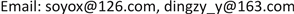1淮海工学院，电子工程学院，江苏 连云港

2江苏苏云医疗器材有限公司，江苏 连云港1. 引言

2. 系统总体方案设计

3. 系统硬件电路设计

4. 牵引电机控制策略设计

4.1. 滑模变结构控制策略设计

y = b x 1 + x 2 (1)

{ x 1 = n r e f − n x 2 = x ˙ 1 = − n ˙ (2)

{ x ˙ 1 = − n ˙ x ˙ 2 = − K T J i ˙ (3)

n = n r e f − c e − b t (4)

f = f e q + f s w (5)

y ˙ = b x ˙ 1 + x ˙ 2 = b x 2 − K T J i ˙ (6)

f e q = J / K T ∫ ( d x 2 ) d t (7)

f = J K T ∫ [ k s + ε s i g n ( s ) + x 2 ] d t (8)

4.2. 无模型预测控制算法设计

u x 0 + u y 0 − 2 u z 0 = − 3 R i z − 3 L s d i z d t + ( e x + e y − 2 e z ) (9)

( 1 + R T s / L s ) [ i z ( k + 1 ) − i z ( k ) ] − [ i z ( k ) − i z ( k − 1 ) ] = T s 3 L s { [ e ( k + 1 ) − e ( k ) ] − [ u ( k + 1 ) ) − u ( k ] } (10)

[ i z ( k + 1 ) − i z ( k ) ] − [ i z ( k ) − i z ( k − 1 ) ] = φ ( k ) × { [ i z ( k ) − i z ( k − 1 ) ] − [ i z ( k − 1 ) − i z ( k − 2 ) ] } (11)

φ ( k ) = [ e ( k + 1 ) − e ( k ) ] − [ u ( k + 1 ) − u ( k ) ] / [ e ( k ) − e ( k − 1 ) ] − [ u ( k ) − u ( k − 1 ) ] (12)

i z ( k + 1 ) = φ ( k ) [ i z ( k ) − 2 i z ( k − 1 ) + i z ( k − 2 ) ] + [ 2 i z ( k ) − i z ( k − 1 ) ] (13)

5. 压力传感器的数据融合算法

6. 实验结果与分析6.1. 无刷直流电机控制策略性能分析

Brushless DC motor related test parameter

R = 1.2 ΩJ = 0.0022 kg∙m2C e = 0.095L − M = 6.3   mH

T L = 2   N ⋅ mk = 0.01 ， ε = 1000.2   sn e = 2500   rpm

6.2. 数据融合结果及性能分析

Fusion result

21.5300.23211.00322.057
2800.2520.9801.996
3400.2490.9572.050
50−20.2331.0332.023
70−30.2181.0042.047

21.5333.00843.99854.997
2823.0324.0014.999
3413.0294.0035.001
50−23.0114.0055.005
70−33.0164.0075.007

6.3. 系统测试与分析

7. 结束语